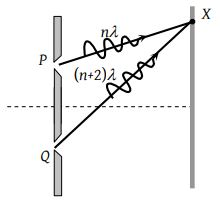The figure shows a double slit experiment P and Q are the slits. The path lengths PX and QX are nλ and (n + 2) λ respectively, where n is a whole number and λ is the wavelength. Taking the central fringe as zero, what is formed at X(1) First bright

(2) First dark

(3) Second bright

(4) Second dark

(3) For brightness, path difference $=n\lambda =2\lambda$

So second is bright.

Difficulty Level:

• 22%
• 18%
• 57%
• 5%